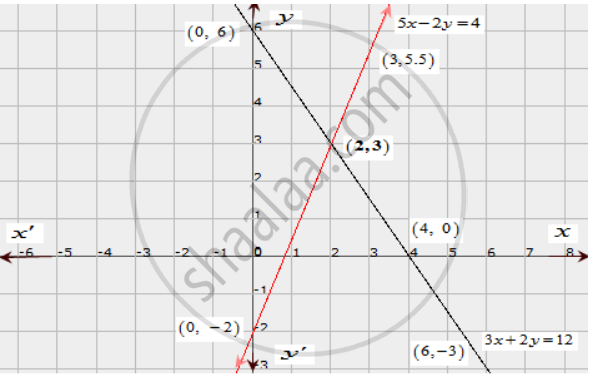Share

# Solve Graphically Each of the Following Systems of Linear Equations. Also, Find the Coordinates of the Points Where the Lines Meet Axis Of Y. 3x + 2y = 12, 5x − 2y = 4 - CBSE Class 10 - Mathematics

ConceptGraphical Method of Solution of a Pair of Linear Equations

#### Question

Solve graphically each of the following systems of linear equations. Also, find the coordinates of the points where the lines meet axis of y.

3x + 2y = 12,

5x − 2y = 4

#### Solution

The given equations are

3x + 2y = 12 ........(i)

5x − 2y = 4 ..........(ii)

The two points satisfying (i) can be listed in a table as,

 x 4 6 y 0 -3

The two points satisfying (ii) can be listed in a table as,

 x 3 2 y 5.5 3

Now, the graph of equations (i) and (ii) can be drawn as,It is seen that the solution of the given system of equations is given by x = 2, y = 3.

Also, it is observed that the lines (i) and (ii) meet the y-axis at the points (0,6) and (0, -2) respectively.

Is there an error in this question or solution?

#### APPEARS IN

Solution Solve Graphically Each of the Following Systems of Linear Equations. Also, Find the Coordinates of the Points Where the Lines Meet Axis Of Y. 3x + 2y = 12, 5x − 2y = 4 Concept: Graphical Method of Solution of a Pair of Linear Equations.
S